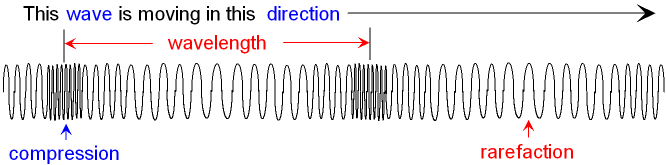gcsescience.com                                       2                                       gcsescience.com

Waves

What is a Longitudinal Wave?

When a longitudinal wave moves through a material,
the particles of the material move backwards and forwards
along the direction in which the wave is travelling.
Below is a picture of a longitudinal wave travelling along a spring.What is the Wavelength of a Longitudinal Wave?

The wavelength of a longitudinal wave can be measured
as the distance between the centre of two compressions.

What is Compression?

Compression is the name given to the region
where the coils of the spring are pushed together.

What is Rarefaction?

Rarefaction (pronounced rair - ree - fac - shun)
is the name given to the region
where the coils of the spring are pulled apart.

It is difficult to show the amplitude and frequency
of a longitudinal wave on a picture.

Examples of longitudinal waves are
P waves from earthquakes and sound waves.

gcsescience.com       Physics Quiz       Index       Wave Quiz       gcsescience.com